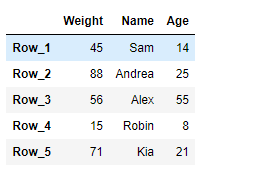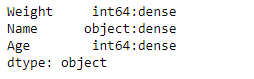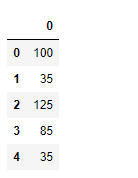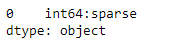Open in App
Not now

# Python | Pandas DataFrame.ftypes

• Last Updated : 20 Feb, 2019

Pandas DataFrame is a two-dimensional size-mutable, potentially heterogeneous tabular data structure with labeled axes (rows and columns). Arithmetic operations align on both row and column labels. It can be thought of as a dict-like container for Series objects. This is the primary data structure of the Pandas.

Pandas` DataFrame.ftypes` attribute return the ftypes (indication of sparse/dense and dtype) in DataFrame. It returns a Series with the data type of each column.

Syntax: DataFrame.ftypes

Parameter : None

Returns : series

Example #1: Use `DataFrame.ftypes` attribute to check if the columns are sparse or dense in the given Dataframe.

 `# importing pandas as pd``import` `pandas as pd`` ` `# Creating the DataFrame``df ``=` `pd.DataFrame({``'Weight'``:[``45``, ``88``, ``56``, ``15``, ``71``],``                   ``'Name'``:[``'Sam'``, ``'Andrea'``, ``'Alex'``, ``'Robin'``, ``'Kia'``],``                   ``'Age'``:[``14``, ``25``, ``55``, ``8``, ``21``]})`` ` `# Create the index``index_ ``=` `[``'Row_1'``, ``'Row_2'``, ``'Row_3'``, ``'Row_4'``, ``'Row_5'``]`` ` `# Set the index``df.index ``=` `index_`` ` `# Print the DataFrame``print``(df)`

Output :Now we will use `DataFrame.ftypes` attribute to check the ftype of the columns in the given dataframe.

 `# check if the column are ``# dense or sparse``result ``=` `df.ftypes`` ` `# Print the result``print``(result)`

Output :As we can see in the output, the `DataFrame.ftypes` attribute has successfully returned a series containing the ftypes of each column in the given dataframe.

Example #2: Use `DataFrame.ftypes` attribute to check if the columns are sparse or dense in the given Dataframe.

 `# importing pandas as pd``import` `pandas as pd`` ` `# Create an array``arr ``=` `[``100``, ``35``, ``125``, ``85``, ``35``]`` ` `# Creating a sparse DataFrame``df ``=` `pd.SparseDataFrame(arr)`` ` `# Print the DataFrame``print``(df)`

Output :Now we will use `DataFrame.ftypes` attribute to check the ftype of the columns in the given dataframe.

 `# check if the column are ``# dense or sparse``result ``=` `df.ftypes`` ` `# Print the result``print``(result)`

Output :As we can see in the output, the `DataFrame.ftypes` attribute has successfully returned the ftype of the given dataframe.

My Personal Notes arrow_drop_up